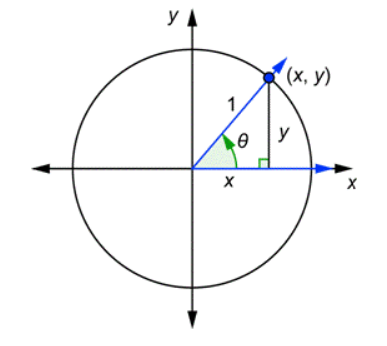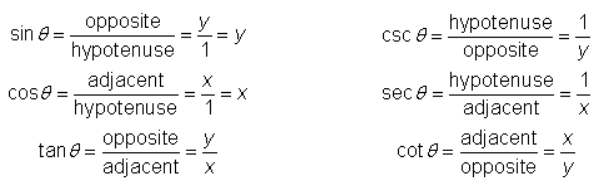# Explain Co-ordinates in trigonometry.

Coordinates and Trigonometric ratios

Suppose we draw any acute angle in standard position together with a unit circle, as seen below.The terminal side of the angle intersects the unit circle at the point (x, y). Let’s write the definitions of the six trigonometric functions and then rewrite them by referring to the triangle above and using the variables x and y.The first equation and the one below it, with the middle steps cut out, tell you:
x = cosθ; y = sinθ

Now you can see that the y-coordinate of this point is always equal to the sine of the angle, and the x-coordinate of this point is always equal to the cosine of the angle.

Now consider any angle θ in standard position together with a unit circle. The terminal side will intersect the circle at some point. Depending on the angle, that point could be in the first, second, third, or fourth quadrant.

Updated on: 10-Oct-2022

27 Views